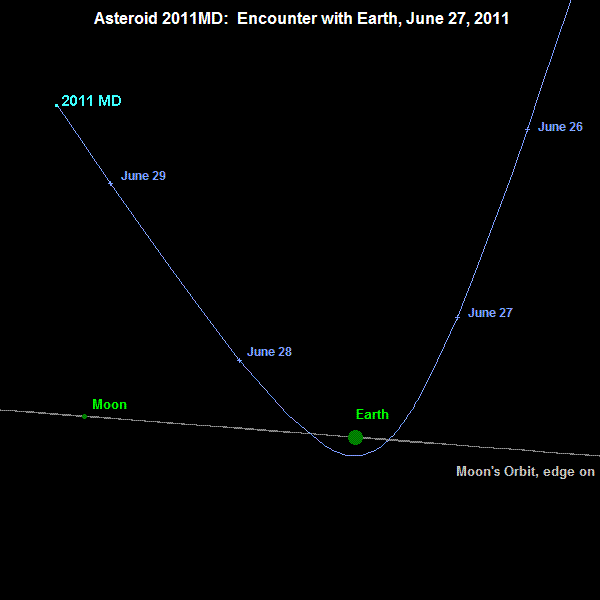# Lets move Earth!

… away from the sun.

I believe that there is a real risk/possibility that at some point humans will attempt some form of geo-engineering to deal with climate change.

One sci-fi idea I have been thinking of concerns moving Earth away from the Sun to reduce the solar constant. I have been wanting to use this idea in teaching as a fun student project. Note: This is not a serious proposal, but just a thought experiment. Other people have thought of this (see links below.)

Q: What would it take to reduce the solar constant by 1% by moving Earth?

(1% is about 4 times the forcing needed to ‘balance’ 4xCO2. See table 2, Kravitz et al. 2013.)

The basic idea is to transfer momentum using gravity assist maneuvers. The way it works is the same idea that was used to sling-shot the Voyager probes far away from Earth. In this way it would be possible to transfer momentum from a close passing object to the Earth.

One idea would be to farm Asteroids with slight nudges far away from Earth in order to harvest some of their momentum. I did a back of envelope calculation that shows it would be hard to do this.

Other ideas have been proposed:

Back of the envelope:

(Note this is a careless BoE calculation. It has not been double checked - it might very well be wrong. If so then please let me know)

Here’s a back of the envelope calculation of what would be required to reduce the solar constant by 1%. This would correspond to ~0.5% increase in the distance to the sun, which in turn would correspond to a 0.25% increase in the velocity of Earth.

Definitions:

U=velocity of Earth

Ma=“Asteroid” mass

Me=Earth mass

The maximum momentum transfer we can get from one sling-shot would be: 2_U_Ma. That gives a velocity change of 2_U_Ma/ME. So to get 0.25% velocity change then we would need to use many asteroid passes with a combined mass of ~ME/80. better calculations can be found here.](http://neo.jpl.nasa.gov/news/news172.html)

Notes on 0.5% and 0.25%:

The (solar constant) amount of radiation received at Earth is proportional to the area of disc with the one Earth radius relative to the area of the sphere with a radius equal to the Sun-Earth distance (rSE). See diagram here. I.e. the solar constant scales with 1/rSE2. Thus a 1.005 increase in rSE corresponds to a 1% decrease in the amount received.

The relationship between orbit radius and velocity can be found here and velocity scales with 1/sqrt(rSE). This gives me the 0.25% velocity change.

Share